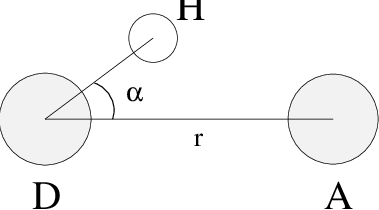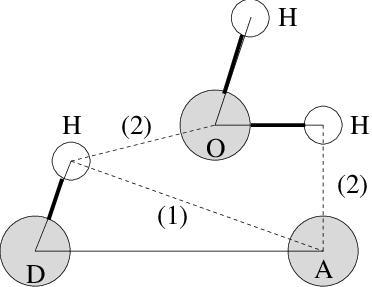# Hydrogen bonds¶

The program gmx hbond analyzes the hydrogen bonds (H-bonds) between all possible donors D and acceptors A. To determine if an H-bond exists, a geometrical criterion is used, see also Fig. 57:
(1)$\begin{split}\begin{array}{rclcl} r & \leq & r_{HB} & = & 0.35~\mbox{nm} \\ \alpha & \leq & \alpha_{HB} & = & 30^o \\ \end{array}\end{split}$Fig. 57 Geometrical Hydrogen bond criterion.

The value of $$r_{HB} = 0.35 \mathrm{nm}$$ corresponds to the first minimum of the RDF of SPC water (see also Fig. 58).

The program gmx hbond analyzes all hydrogen bonds existing between two groups of atoms (which must be either identical or non-overlapping) or in specified donor-hydrogen-acceptor triplets, in the following ways:Fig. 58 Insertion of water into an H-bond. (1) Normal H-bond between two residues. (2) H-bonding bridge via a water molecule.

• Donor-Acceptor distance ($$r$$) distribution of all H-bonds

• Hydrogen-Donor-Acceptor angle ($$\alpha$$) distribution of all H-bonds

• The total number of H-bonds in each time frame

• The number of H-bonds in time between residues, divided into groups $$n$$-$$n$$+$$i$$ where $$n$$ and $$n$$+$$i$$ stand for residue numbers and $$i$$ goes from 0 to 6. The group for $$i=6$$ also includes all H-bonds for $$i>6$$. These groups include the $$n$$-$$n$$+$$3$$, $$n$$-$$n$$+$$4$$ and $$n$$-$$n$$+$$5$$ H-bonds, which provide a measure for the formation of $$\alpha$$-helices or $$\beta$$-turns or strands.

• The lifetime of the H-bonds is calculated from the average over all autocorrelation functions of the existence functions (either 0 or 1) of all H-bonds:

(2)$C(\tau) ~=~ \langle s_i(t)~s_i (t + \tau) \rangle$
• with $$s_i(t) = \{0,1\}$$ for H-bond $$i$$ at time $$t$$. The integral of $$C(\tau)$$ gives a rough estimate of the average H-bond lifetime $$\tau_{HB}$$:

(3)$\tau_{HB} ~=~ \int_{0}^{\infty} C(\tau) d\tau$
• Both the integral and the complete autocorrelation function $$C(\tau)$$ will be output, so that more sophisticated analysis (e.g. using multi-exponential fits) can be used to get better estimates for $$\tau_{HB}$$. A more complete analysis is given in ref. 173; one of the more fancy option is the Luzar and Chandler analysis of hydrogen bond kinetics 174, 175.

• An H-bond existence map can be generated of dimensions # H-bonds$$\times$$# frames. The ordering is identical to the index file (see below), but reversed, meaning that the last triplet in the index file corresponds to the first row of the existence map.

• Index groups are output containing the analyzed groups, all donor-hydrogen atom pairs and acceptor atoms in these groups, donor-hydrogen-acceptor triplets involved in hydrogen bonds between the analyzed groups and all solvent atoms involved in insertion.